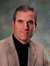Addition (often signified by the plus symbol "+") is one of the four basic operations of arithmetic, with the others being subtraction, multiplication and division. The addition of two whole numbers is the total amount of those quantities combined. For example, in the picture on the right, there is a combination of three apples and two apples together, making a total of five apples. This observation is equivalent to the mathematical expression "3 + 2 = 5" i.e., "3 add 2 is equal to 5".

Besides counting fruits, addition can also represent combining other physical objects. Using systematic genera
...more## Featured News & Interviews